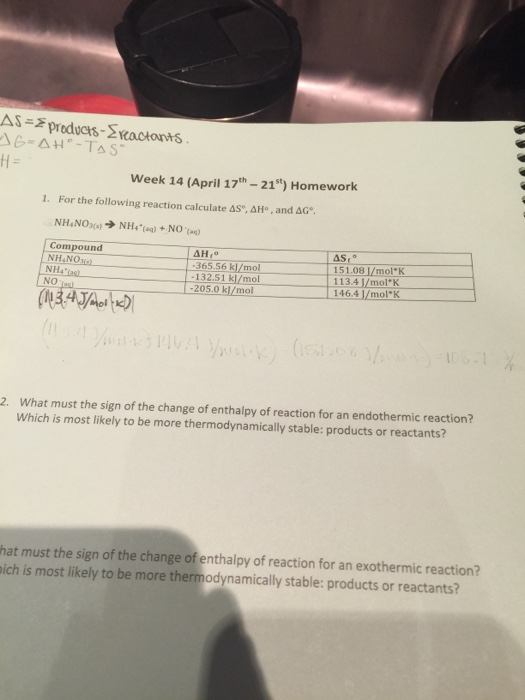# Question & Answer: For the following reaction calculate Delta S degree, Delta G degree. NH_4NO_3 (s) rightarrow…..For the following reaction calculate Delta S degree, Delta G degree. NH_4NO_3 (s) rightarrow NH^+_4 (aq) + NO^- (aq) What must the sign of the change of enthalpy of reaction for an endothermic reaction? Which is most likely to be more thermodynamically stable: products or reactants? What must the sign of the change of enthalpy of reaction for an exothermic reaction? Which is most likely to be more thermodynamically stable: products or reactants?

Ans. #1. dS0 of reaction = (sum of dS0f of products) – (sum of dS0f of reactant)

Or, dS0 = (113.4 J mol-1 K-1 + 146.4 J mol-1 K-1) – 151.08 J mol-1 K-1

Don't use plagiarized sources. Get Your Custom Essay on
Question & Answer: For the following reaction calculate Delta S degree, Delta G degree. NH_4NO_3 (s) rightarrow…..
GET AN ESSAY WRITTEN FOR YOU FROM AS LOW AS \$13/PAGE

Or, dS0 = 259.80 J mol-1 K-1 – 151.08 J mol-1 K-1

Hence, dS0 = 108.72 J mol-1 K-1 = 0.10872 kJ mol-1 K-1

#2. dH0 of reaction = (sum of dH0f of products) – (sum of dH0f of reactants)

Or, dH0 = (-132.51 kJ mol-1 – 205.0 kJ mol-1) – (- 365.56 kJ mol-1)

Or, dH0 = – 337.51 kJ mol-1 + 365.56 kJ mol-1

Hence, dH0 = 28.05 kJ mol-1

#3. dG0 = dH0 – T (dS)

Note that standard temperature in chemistry is taken to be 0.00C = 273.15 K

Putting the values in above equation-

dG0 = (28.05 kJ mol-1) – 273.15 K x (0.10872 kJ mol-1 K-1)

Or, dG0 = 28.05 kJ mol-1 – 29.70 kJ mol-1

Hence, dG0 = – 1.65 kJ mol-1